﻿ Maya> other>Finding Distances In Maya

# ﻿Finding Distances In Maya

﻿

This tutorial teaches you how to find distances in Maya.

Go to create>measure tools>distance tool. Now click anywhere on the grid twice to define two distance points.

When you're done, it will create two locators and the distance node. The distance node displays the distance of Maya units in the view port.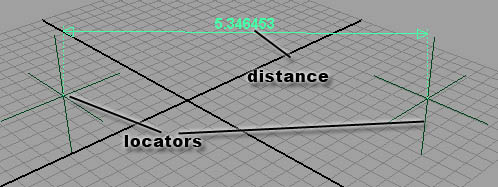Now let's see how it works. Select the distance node and go to window>attributes editor, then go to the shape node.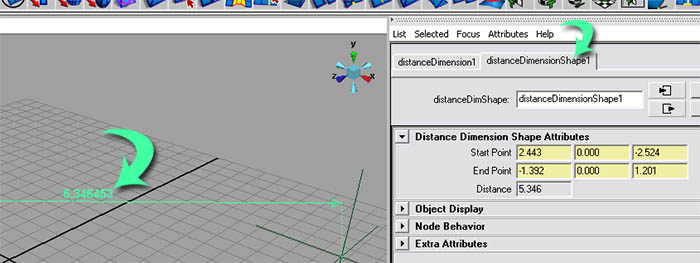Here you'll find the start and end point attributes. These attributes contain the X, Y, and Z position of both of the locators. These attributes are connected to the locators, so, if you were to move one of the locators, the distance would update. The attribute distance is the output and the distance between the points above.

If you want to define the distance between two objects (models), there's a quick way of doing it.

Step one - Create a new scene. For an example, create two cubes and position them side-by-side.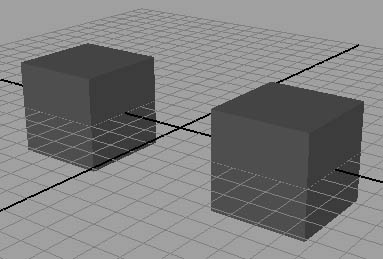Step two - Now use the distance tool and create two locators (distance points). It doesn't matter where the are.

Step three - Go to the animation menu set.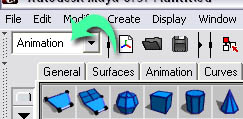Step four - Select one of the cubes, hold the shift key, and select one of the locators; this order is important, because we want the locator connected to the cube, not the other way around. Then go to constrain>point.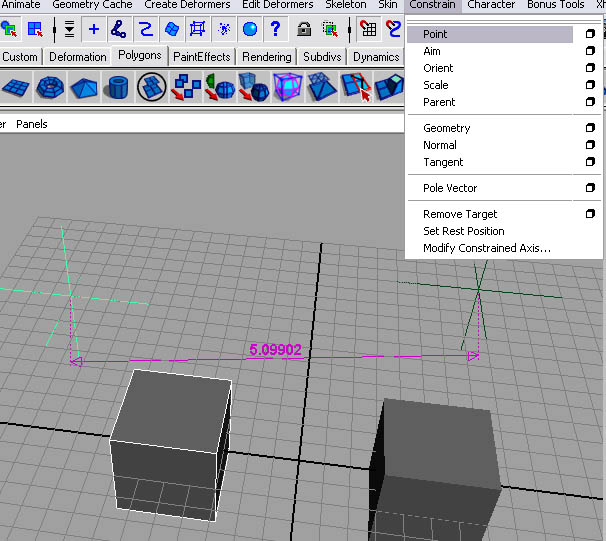Step five - Select the other cube and the other locator and go to constrain>point.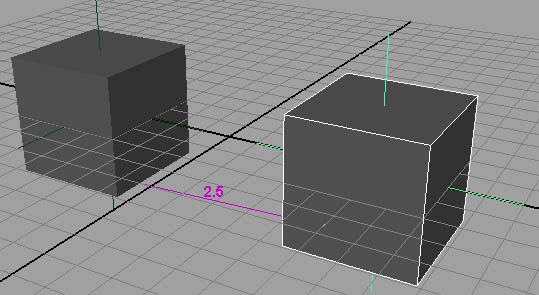Now you have the distance between those two objects. This measurement is based on the objects pivot point (not the surfaces), which, by default, is in the center of the object. If you have a problem using this technique, try selecting the model and going to modify>center pivot.

Good luck.Home Tutorials About the Author Site Map